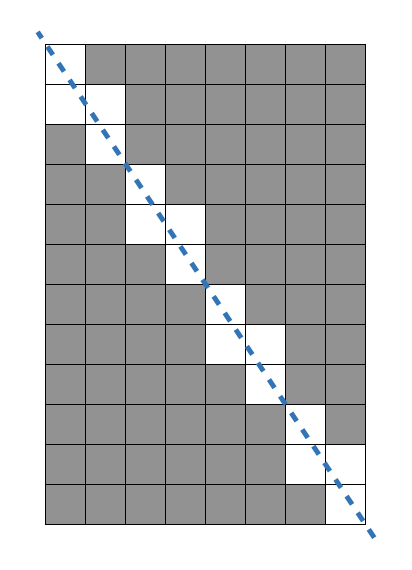###### Description

There is a `W`cm x `H`cm paper grid. Each grid is 1cm x 1cm. Suppose that you were going to cut out and use each of the 1cm x 1cm square grid, but someone cut the paper diagonally so you now have two right-triangle paper pieces. You don’t have another piece of grid paper, so you decide to use the two triangle paper pieces.
Given W and H, write a `solution` function that returns the number of 1 x 1 squares that you can use.

##### Constraints
• `W` and `H` are integers. 1 ≤ `W`, `H` ≤ 100,000,000

#### Example

W H result
8 12 80

Example #1

The size of the paper board is 8 x 12. With this rectangle, you can make 96 squares. But, by cutting the paper board diagonally, 16 squares turns into 32 triangles. So you can get (96 - 16 = 80) squares.Result
Result of [Run Test] or [Submit] will be displayed here